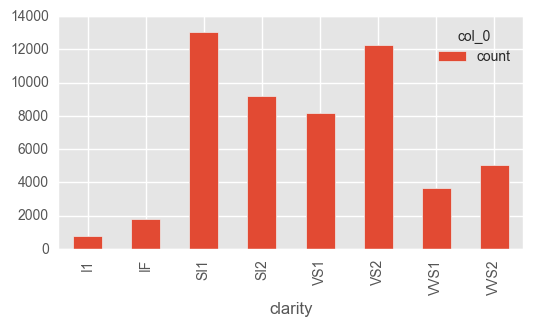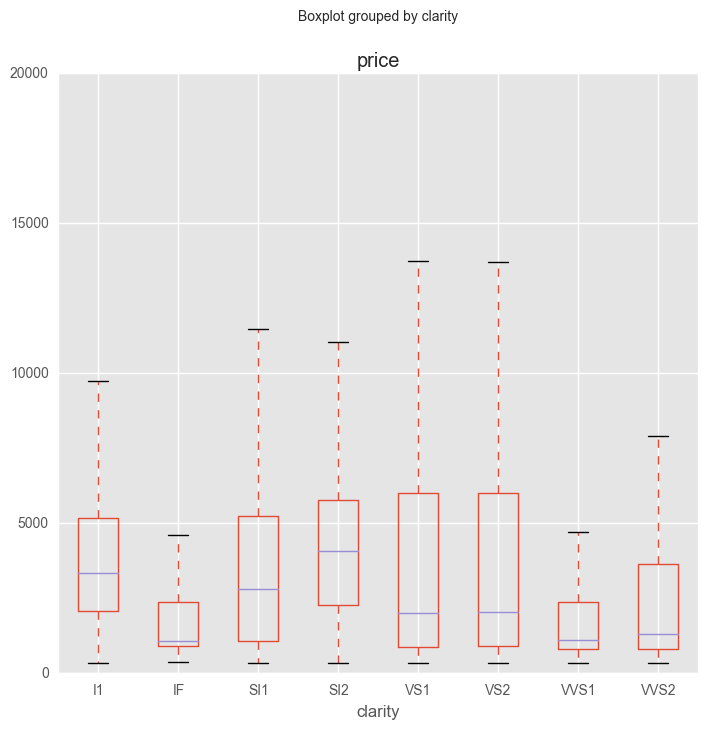Data Analysis Resources

# Visualise Categorical Variables in PythonIt is crucial to learn the methods of dealing with categorical variables as categorical variables are known to hide and mask lots of interesting information in a data set. A categorical variable identifies a group to which the thing belongs. You could categorise persons according to their race or ethnicity, cities according to their geographic location, or companies according to their industry. However, I have always found a challenge to visualise categorical variables in python.

In this article, I use the ggplot2 diamond dataset to explore various techniques while visualising categorical variables in python.

If you find this article helpful or know of other methods which work well with categorical variables? Please share your thoughts in the comments section below. I’d love to hear you.

## Visualise Categorical Variables in Python  using Univariate Analysis

At this stage, we explore variables one by one.  For categorical variables, we’ll use a frequency table to understand the distribution of each category. It is also used to highlight missing and outlier values.We can also read as a percentage of values under each category. It can be measured using two metrics, Count and Count% against each category. A bar chart can be used as visualisation.

One-Way Tables

Create frequency tables (also known as crosstabs) in pandas using the pd.crosstab() function. The function takes one or more array-like objects as indexes or columns and then constructs a new DataFrame of variable counts based on the supplied arrays.

Let’s make a one-way table of the clarity variable. Even these simple one-way tables give us some useful insight: we immediately get a sense of the distribution of records across the categories.

clarity variable. Even these simple one-way tables give us some useful insight: we immediately get a sense of the distribution of records across the categories.

In :
```my_tab = pd.crosstab(index = train["clarity"],  # Make a crosstab
columns="count")      # Name the count column

my_tab.plot.bar()
```
Out:
`<matplotlib.axes._subplots.AxesSubplot at 0x2c373671b00>`Since the crosstab function produces DataFrames, the DataFrame operations work on crosstabs.

```In :
print (my_tab.sum(), "\n")   # Sum the counts

print (my_tab.shape, "\n")   # Check number of rows and cols

my_tab.iloc[1:7]             # Slice rows 1-6
```
```col_0
count    53940
dtype: int64

(8, 1)

```
Out:
col_0 count
clarity
IF 1790
SI1 13065
SI2 9194
VS1 8171
VS2 12258
VVS1 3655

One of the most useful aspects of frequency tables is that they allow you to extract the proportion of the data that belongs to each category. With a one-way table, you can do this by dividing each table value by the total number of records in the table:

In :
```my_tab/my_tab.sum()
```
Out:
col_0 count
clarity
I1 0.013737
IF 0.033185
SI1 0.242214
SI2 0.170449
VS1 0.151483
VS2 0.227253
VVS1 0.067760
VVS2 0.093919

## Visualise Categorical Variables in Python using Bivariate Analysis

Bivariate Analysis finds out the relationship between two variables. Here, we look for association and disassociation between variables at a pre-defined significance level.

Categorical & Continous: To find the relationship between categorical and continuous variables, we can use Boxplots

Boxplots are another type of univariate plot for summarising distributions of numeric data graphically. Let’s make a boxplot of carat using the pd.boxplot() function:

The central box of the boxplot represents the middle 50% of the observations, the central bar is the median and the bars at the end of the dotted lines (whiskers) encapsulate the great majority of the observations. Circles that lie beyond the end of the whiskers are data points that may be outliers.

```In :
train.boxplot(column="price",        # Column to plot
by= "clarity",         # Column to split upon
figsize= (8,8))        # Figure size
```
Out:
`<matplotlib.axes._subplots.AxesSubplot at 0x2801cdfe048>`The boxplot above is curious: we’d expect diamonds with better clarity to fetch higher prices and yet diamonds on the highest end of the clarity spectrum (IF = internally flawless) actually have lower median prices than low clarity diamonds!

#### Categorical & Categorical: To find the relationship between two categorical variables, we can use following methods:

• Two-way table: We can start analysing the relationship by creating a two-way table of count and count%. The rows represent the category of one variable and the columns represent the categories of the other variable. We show count or count% of observations available in each combination of row and column categories.
• Stacked Column Chart: This method is more of a visual form of a Two-way table.
```In :
#two-way table
grouped = train.groupby(['cut','clarity'])
grouped.size()```
Out:
```cut        clarity
Fair       I1          210
IF            9
SI1         408
SI2         466
VS1         170
VS2         261
VVS1         17
VVS2         69
Good       I1           96
IF           71
SI1        1560
SI2        1081
VS1         648
VS2         978
VVS1        186
VVS2        286
Ideal      I1          146
IF         1212
SI1        4282
SI2        2598
VS1        3589
VS2        5071
VVS1       2047
VVS2       2606
IF          230
SI1        3575
SI2        2949
VS1        1989
VS2        3357
VVS1        616
VVS2        870
Very Good  I1           84
IF          268
SI1        3240
SI2        2100
VS1        1775
VS2        2591
VVS1        789
VVS2       1235
dtype: int64```

#### Two-Way Tables

Two-way frequency tables, also called contingency tables, are tables of counts with two dimensions where each dimension is a different variable. Two-way tables can give you insight into the relationship between two variables. To create a two-way table, pass two variables to the pd.crosstab() function instead of one:

```clarity_color_table = pd.crosstab(index=train["clarity"],
columns=train["color"])

clarity_color_table
```
Out:
color D E F G H I J
clarity
I1 42 102 143 150 162 92 50
IF 73 158 385 681 299 143 51
SI1 2083 2426 2131 1976 2275 1424 750
SI2 1370 1713 1609 1548 1563 912 479
VS1 705 1281 1364 2148 1169 962 542
VS2 1697 2470 2201 2347 1643 1169 731
VVS1 252 656 734 999 585 355 74
VVS2 553 991 975 1443 608 365 131
In :
```clarity_color_table.plot(kind="bar",
figsize=(8,8),
stacked=True)
```
Out:
`<matplotlib.axes._subplots.AxesSubplot at 0x2801e2975f8>`## 3 thoughts on “Visualise Categorical Variables in Python”

1.Amin Ghaderi says:

very interesting,

thanks!

2.bear says:

good work

3.András Novoszáth says:

Quite nice!